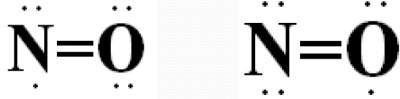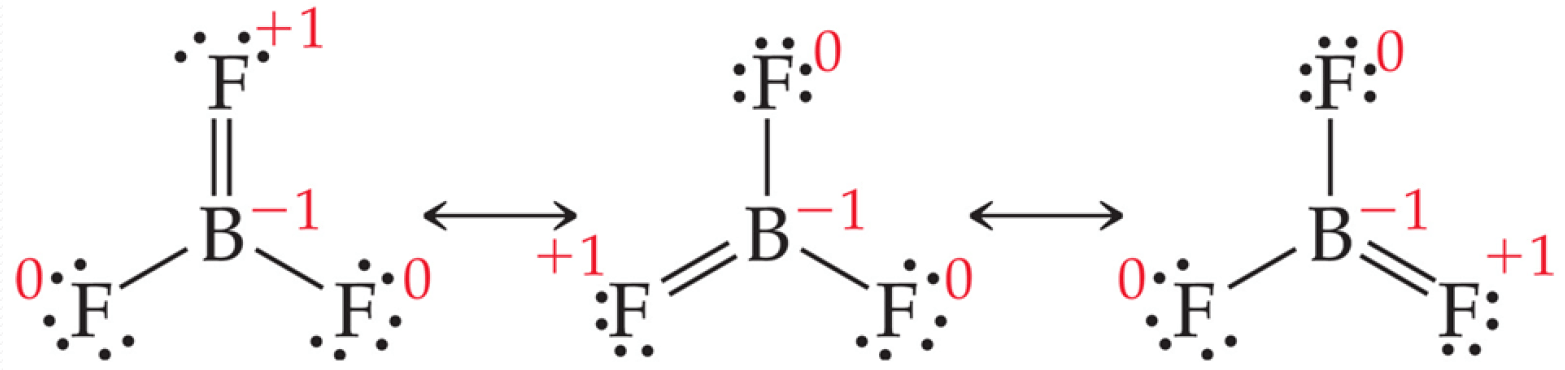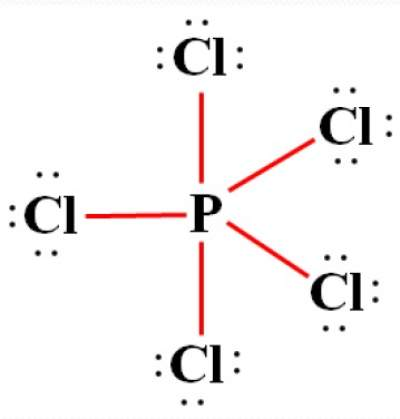# Exceptions to the Octet Rule

## Reminder about the Octet Rule

"Atoms tend to gain, loseor shareelectrons until they are surrounded by 8 valence electrons"

Exceptions to the Octet Rule
• Atoms with a total odd number of electrons
• Less than Octet
• More than Octet

### Example on Atoms with a total odd number of electrons: NO Molecule

Total number of valence electrons = 5 (of N) + 6 (of O) = 11 (odd number)### Example on Less than Octet: BF3 MoleculeDespite these correct resonance structures, they are not possible due to the unacceptable formal charge on F.

### Example on More than Octet: PCl5 Molecule• Where the central atom (P) is surrounded by more than octet (8).
• This is observed in elements where the 3rd shell(and beyond) are being filled
• Here, the outermost configuration of P is: 3s2, 3p3, 3d0
• Such elements exceed octet by accommodating more electrons in their empty d orbitals